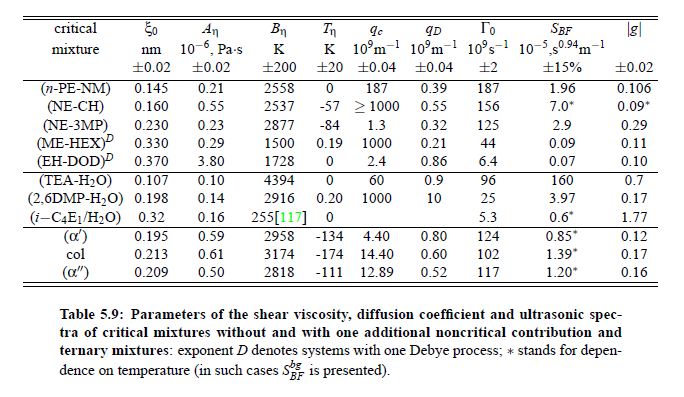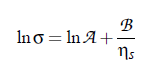### Article Index

Parameters which have been obtained from ultrasonic spectrometry, dynamic light scattering and shear viscosity are presented in Table (5.9). It is a fascinating aspect of the dynamic scaling theory of ultrasonic attenuation that, due to scaling of frequency data of different critical mixtures fall on one scaling function.  However, the most curious speciﬁc system parameter is the characteristic relaxation rate amplitude Γ_0, which according to Bhattacharjee-Ferrell theory, corresponds with the mutual diffusion coefﬁcient D and the ﬂuctuation correlation length ξ.  In Table (5.9) parameters Γ_0  and ξ_0  are listed for various binary mixtures with critical demixing point. The isobutoxyethanol-water system exhibits by far the smallest amplitude Γ_0 in the relaxation rate of order parameter ﬂuctuations. In comparison, with the system n-pentanol-nitromethane, Γ_0 is 35 times larger.  Assuming, that the life time of ﬂuctuations τξ  = Γ_0^−1, as inverse characteristic relaxation rate reﬂects intermolecular properties as well the geometry of considered components the strong variation of Γ_0  of various liquids can be understood. In addition, due to the Coulombic interactions, relaxation from a local nonequilibrium distribution of electrical charges into thermal equilibrium will involve extensive redistribution of ions in ionic solutions and may, therefore, proceed with a smaller relaxation rate than a molecular liquid mixture at the same reduced temperature.  A quantity, which may be taken to summarize the above mentioned molecular properties, is the surface tension σ. If considering critical ﬂuctuations, reﬂected by the ﬂuctuation relaxation rate Γ_0  , to depend on the  surface  tension,  a correlation  between  both  quantities  should  exist.Based  on  this idea, Khabibullaev and Mirzaev found a correlation between sound attenuation and surface tension.  One of the most important parameters in the determination of the characteristic relaxation rate is the viscosity. The ﬁrst relation between surface tension and viscosity, has been presented by Pelofsky  as a relation between these two thermophysical properties:where A and B are constants, σ is the surface tension, and ηs  the viscosity.  According to this equation, this empirical expression can be applied for pure and mixed components. Several ﬂuids were shown to follow these relations: n-alkanes, benzene, toluene, xylenes, phenol and other aromatics, n-alcohols in the range, water and some aqueous solutions.